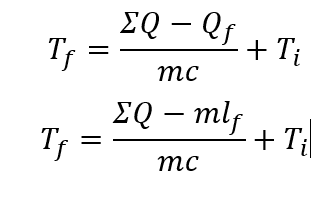# Final temperature of water

ayans2495
Homework Statement:
An insulated container holding 4.55kg of ice at 0.00 degrees Celsius has 2.65 MJ of work done on it while a heater provides 14600 J of heat to the ice. If the latent heat of fusion of ice is 3.34 x 10^5 Jkg^-1, calculate the final temp of the water. Assume that the increase in internal energy is first due to an increase in the potential energy then an increase in the kinetic energy.
Relevant Equations:
Q=mcΔT
Q=ml
ΔU = Q - WHomework Helper
Hi,
1. Where is your work ?
2. What do the symbols stand for ?
3. What is your question ?

Did you read the guidelines ?

•Chestermiller
rsk
Calculate how much work/energy is given to the ice altogther.

Calculate how much of this is needed to melt the ice. This is the "due to an increase in the potential energy" part of the question. You have the equation and the data you need.

Once the ice has melted, the rest of that work/energy goes into raising the kinetic energy, ie the temperature of the water. Again, you have the equation, although the shc for water isn't stated in what you've give us. You can easily find it online but I'd suggest using whatever value you've been given in class as the value is quoted with varying degrees of precision and it's probably best to use the one your teacher/lecturer/textbook expects.